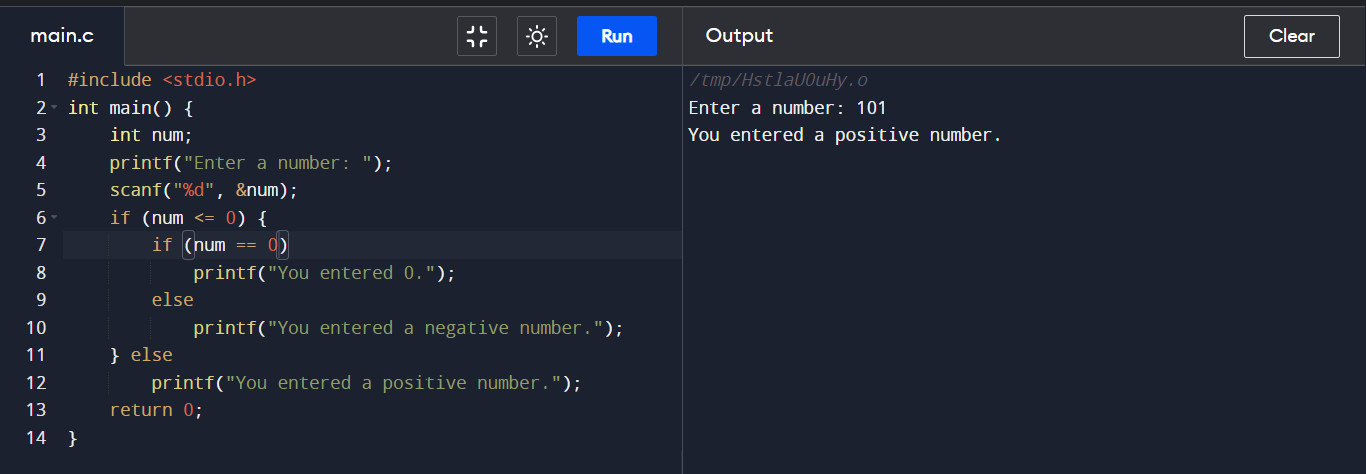## C Program to check Number (Positive or Negative or Zero)

How to check if a Number is Positive or Negative or Zero in C? By this following program we can do this. Here we used "if-else" statements to check the Number type.

#### Positive or Negative checking by C :

``````
#include <stdio.h>
int main() {
int num;
printf("Enter a number: ");
scanf("%d", &num);
if (num <= 0) {
if (num == 0)
printf("You entered 0.");
else
printf("You entered a negative number.");
} else
printf("You entered a positive number.");
return 0;
}

``````

#### Output :